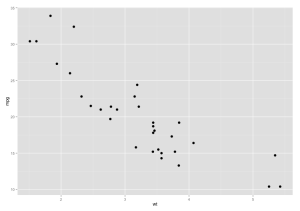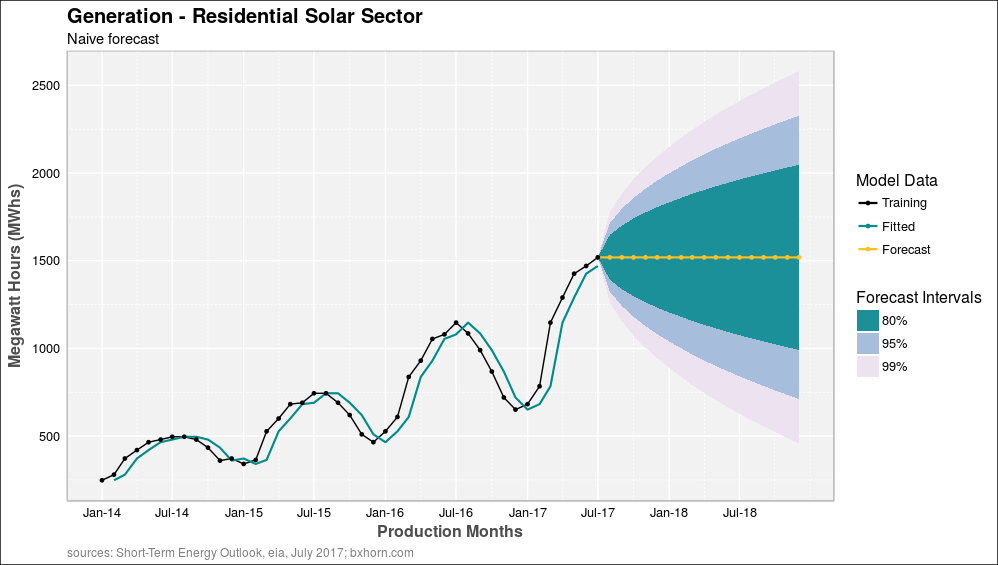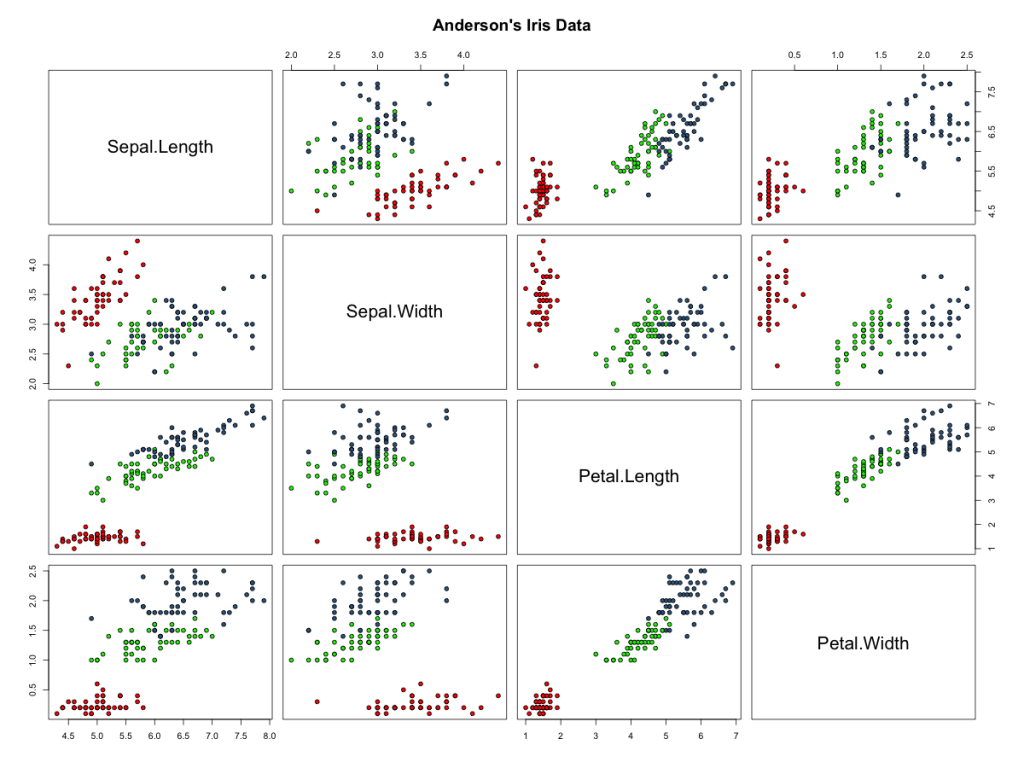# Category Archives: ggplot2

## Scatter Plots (ggplot)

Scatter plots in ggplot are simple to construct and can utilize many format options.

#### Data

The mtcars data frame ships with R and was extracted from the 1974 US Magazine Motor Trend.  The data compares fuel consumption and 10 aspects of automobile design and performance for 32 automobiles (1973–74 models).

#### Basic Scatter Plot Syntax#### Aesthetics

Scatter plot aesthetics are used to control selected x and y data, color (by name),  point shape (1 thru 24), alpha level (or transparency), point size, and point fill.

Posted in ggplot2 | Comments Off on Scatter Plots (ggplot)

## Text (ggplot)

Posted in ggplot2 | Comments Off on Text (ggplot)

## Plotting Forecast Data Objects Using ggplotRobert Hyndman is the author of the forecast package in R. I’ve been using the package for long-term time series forecasts. The package comes with some built in methods for plotting forecast data objects in R that Ive wanted to customize for improved clarity and presentation.  The following article achieves that goal and shares two scripts for plotting forecast data objects using ggplot.

Posted in Data Science, ggplot2, Modeling, R Programming | Comments Off on Plotting Forecast Data Objects Using ggplot

## Correlation Plots in R

The standard function for correlation plots in R is pairs(), which generates a matrix of scatter plots based on all pairwise combinations of variables in a data object.  The standard graph looks something like this after a little color enhancement:”Click to enlarge

The code behind this plot is simple:

Posted in Data Science, ggplot2, R Graphics, R Programming | Comments Off on Correlation Plots in R